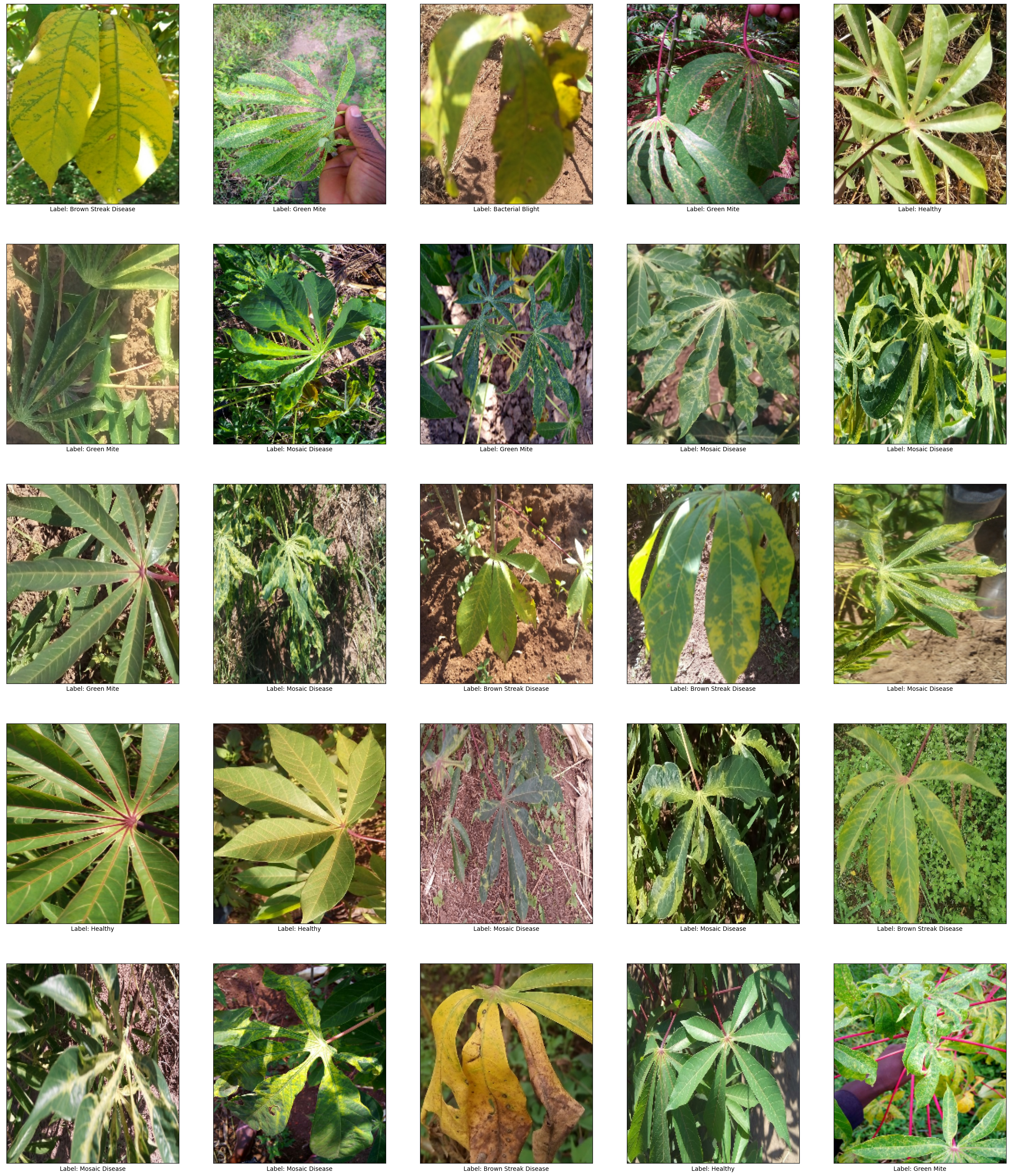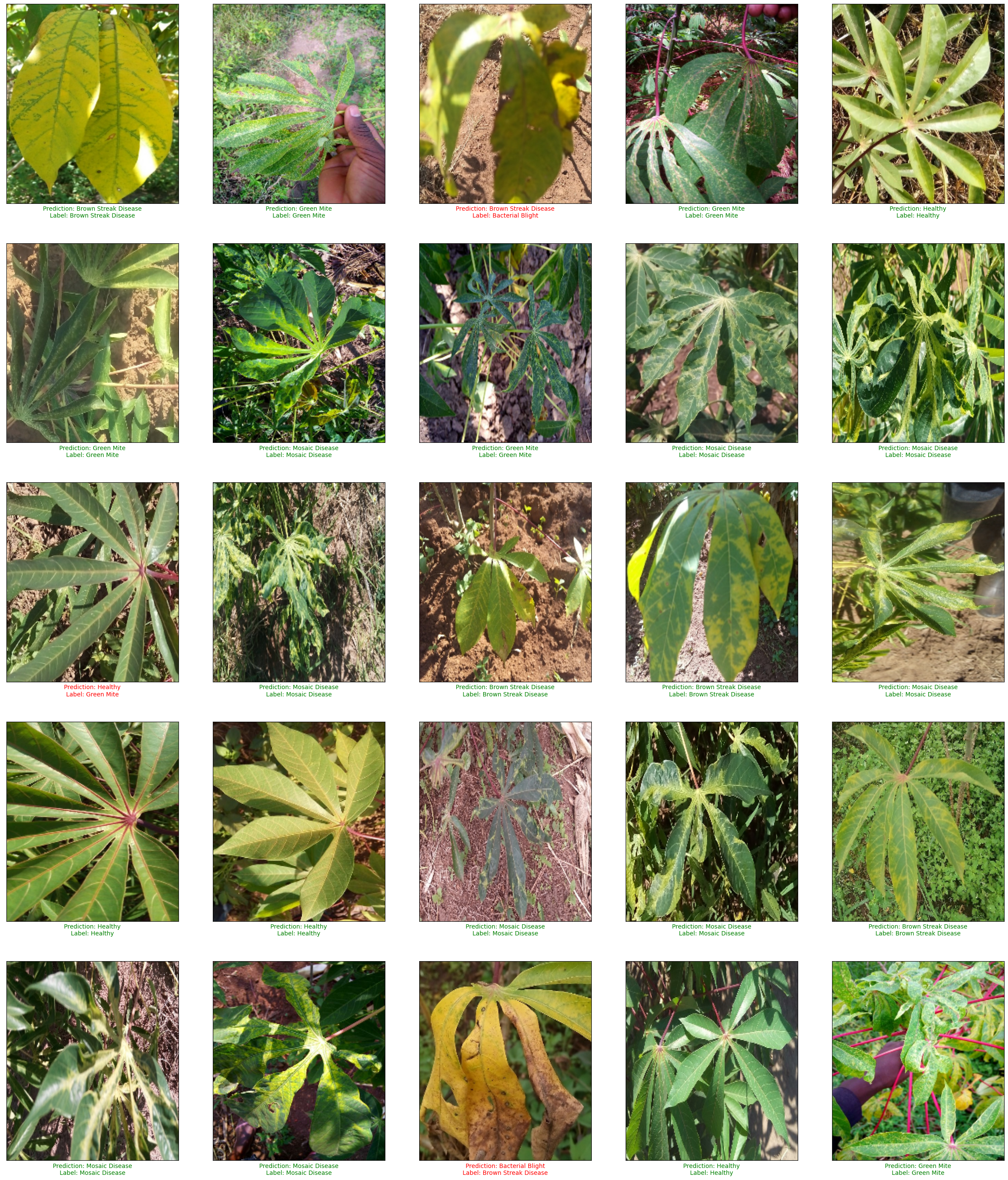Thanks for tuning in to Google I/O. View all sessions on demand

# CropNet: Cassava Disease Detection

This notebook shows how to use the CropNet cassava disease classifier model from TensorFlow Hub. The model classifies images of cassava leaves into one of 6 classes: bacterial blight, brown streak disease, green mite, mosaic disease, healthy, or unknown.

This colab demonstrates how to:

• Load the cassava dataset from TensorFlow Datasets (TFDS)
• Classify images of cassava leaves into 4 distinct cassava disease categories or as healthy or unknown.
• Evaluate the accuracy of the classifier and look at how robust the model is when applied to out of domain images.

## Imports and setup

````pip install matplotlib==3.2.2`
```
``````import numpy as np
import matplotlib.pyplot as plt

import tensorflow as tf
import tensorflow_datasets as tfds
import tensorflow_hub as hub
``````

## Dataset

Let's load the cassava dataset from TFDS

``````dataset, info = tfds.load('cassava', with_info=True)
``````

Let's take a look at the dataset info to learn more about it, like the description and citation and information about how many examples are available

``````info
``````
```tfds.core.DatasetInfo(
name='cassava',
full_name='cassava/0.1.0',
description="""
Cassava consists of leaf images for the cassava plant depicting healthy and
four (4) disease conditions; Cassava Mosaic Disease (CMD), Cassava Bacterial
Blight (CBB), Cassava Greem Mite (CGM) and Cassava Brown Streak Disease (CBSD).
Dataset consists of a total of 9430 labelled images.
The 9430 labelled images are split into a training set (5656), a test set(1885)
and a validation set (1889). The number of images per class are unbalanced with
the two disease classes CMD and CBSD having 72% of the images.
""",
homepage='https://www.kaggle.com/c/cassava-disease/overview',
data_path='gs://tensorflow-datasets/datasets/cassava/0.1.0',
file_format=tfrecord,
dataset_size=Unknown size,
features=FeaturesDict({
'image': Image(shape=(None, None, 3), dtype=uint8),
'image/filename': Text(shape=(), dtype=string),
'label': ClassLabel(shape=(), dtype=int64, num_classes=5),
}),
supervised_keys=('image', 'label'),
disable_shuffling=False,
splits={
'test': <SplitInfo num_examples=1885, num_shards=4>,
'train': <SplitInfo num_examples=5656, num_shards=8>,
'validation': <SplitInfo num_examples=1889, num_shards=4>,
},
citation="""@misc{mwebaze2019icassava,
title={iCassava 2019Fine-Grained Visual Categorization Challenge},
author={Ernest Mwebaze and Timnit Gebru and Andrea Frome and Solomon Nsumba and Jeremy Tusubira},
year={2019},
eprint={1908.02900},
archivePrefix={arXiv},
primaryClass={cs.CV}
}""",
)
```

The cassava dataset has images of cassava leaves with 4 distinct diseases as well as healthy cassava leaves. The model can predict all of these classes as well as sixth class for "unknown" when the model is not confident in it's prediction.

``````# Extend the cassava dataset classes with 'unknown'
class_names = info.features['label'].names + ['unknown']

# Map the class names to human readable names
name_map = dict(
cmd='Mosaic Disease',
cbb='Bacterial Blight',
cgm='Green Mite',
cbsd='Brown Streak Disease',
healthy='Healthy',
unknown='Unknown')

print(len(class_names), 'classes:')
print(class_names)
print([name_map[name] for name in class_names])
``````
```6 classes:
['cbb', 'cbsd', 'cgm', 'cmd', 'healthy', 'unknown']
['Bacterial Blight', 'Brown Streak Disease', 'Green Mite', 'Mosaic Disease', 'Healthy', 'Unknown']
```

Before we can feed the data to the model, we need to do a bit of preprocessing. The model expects 224 x 224 images with RGB channel values in [0, 1]. Let's normalize and resize the images.

``````def preprocess_fn(data):
image = data['image']

# Normalize [0, 255] to [0, 1]
image = tf.cast(image, tf.float32)
image = image / 255.

# Resize the images to 224 x 224
image = tf.image.resize(image, (224, 224))

data['image'] = image
return data
``````

Let's take a look at a few examples from the dataset

``````batch = dataset['validation'].map(preprocess_fn).batch(25).as_numpy_iterator()
examples = next(batch)
plot(examples)
``````## Model

Let's load the classifier from TF Hub and get some predictions and see the predictions of the model is on a few examples

``````classifier = hub.KerasLayer('https://tfhub.dev/google/cropnet/classifier/cassava_disease_V1/2')
probabilities = classifier(examples['image'])
predictions = tf.argmax(probabilities, axis=-1)
``````
```WARNING:tensorflow:Please fix your imports. Module tensorflow.python.training.tracking.data_structures has been moved to tensorflow.python.trackable.data_structures. The old module will be deleted in version 2.11.
WARNING:tensorflow:Please fix your imports. Module tensorflow.python.training.tracking.data_structures has been moved to tensorflow.python.trackable.data_structures. The old module will be deleted in version 2.11.
```
``````plot(examples, predictions)
``````## Evaluation & robustness

Let's measure the accuracy of our classifier on a split of the dataset. We can also look at the robustness of the model by evaluating its performance on a non-cassava dataset. For image of other plant datasets like iNaturalist or beans, the model should almost always return unknown.

### Parameters

``````def label_to_unknown_fn(data):
data['label'] = 5  # Override label to unknown.
return data
``````
``````# Preprocess the examples and map the image label to unknown for non-cassava datasets.
dataset_description = DATASET
if DATASET != 'cassava':
ds = ds.map(label_to_unknown_fn)
dataset_description += ' (labels mapped to unknown)'
ds = ds.batch(BATCH_SIZE)

# Calculate the accuracy of the model
metric = tf.keras.metrics.Accuracy()
for examples in ds:
probabilities = classifier(examples['image'])
predictions = tf.math.argmax(probabilities, axis=-1)
labels = examples['label']
metric.update_state(labels, predictions)

print('Accuracy on %s: %.2f' % (dataset_description, metric.result().numpy()))
``````
```Accuracy on cassava: 0.88
```